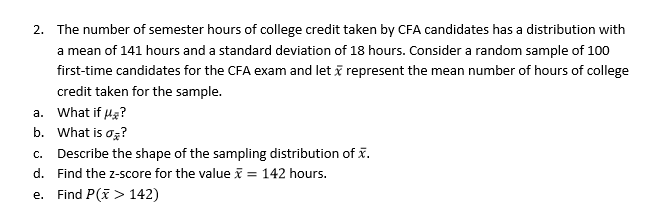# The number of semester hours of college credit taken by CFA candidates has a distribution witha mean of 141 hours and a standard deviation of 18 hours. Consider a random sample of 100first-time candidates for the CFA exam and letcredit taken for the sample.2.represent the mean number of hours of collegea. what if μ?b. what is ơi?c. Describe the shape of the sampling distribution of x.d. Find the z-score for the value 142 hours.e. Find P(> 142)

Question
6 views

Parts D and Ehelp_outlineImage TranscriptioncloseThe number of semester hours of college credit taken by CFA candidates has a distribution with a mean of 141 hours and a standard deviation of 18 hours. Consider a random sample of 100 first-time candidates for the CFA exam and let credit taken for the sample. 2. represent the mean number of hours of college a. what if μ? b. what is ơi? c. Describe the shape of the sampling distribution of x. d. Find the z-score for the value 142 hours. e. Find P(> 142) fullscreen
check_circle

Step 1

Solution:

d.Finding the z-score for the value x-bar=142 hours.

If a random sample of size n is taken from a population having mean µ and standard deviation σ then, the z-score is defined as,

Step 2

Let the random variable X denote the number of semester hours of college credit taken by candidates. The mean µ is 141 hours and the standard deviation σ is 18 hours. A random sample (n) of 100 candidates is selected. Thus, the z-score is,

Step 3

Thus, the z-score for the value x-bar=142 hours is 0.56.

e.Finding P(x-bar>142).

The required probability is ca...

### Want to see the full answer?

See Solution

#### Want to see this answer and more?

Solutions are written by subject experts who are available 24/7. Questions are typically answered within 1 hour.*

See Solution
*Response times may vary by subject and question.
Tagged in

### Other